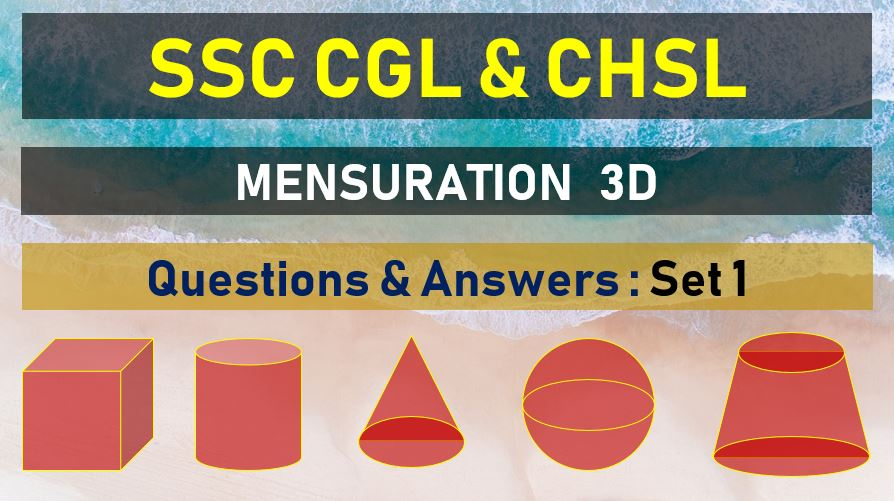Monday, September 27, 2021
Home > mensuration > SSC CGL Surface Area and Volume Questions Answers Set 1

# SSC CGL Surface Area and Volume Questions Answers Set 1SSC Mensurations 3D
Surface Area & Volume Questions Sets
Set 1Set 2Set 3Set 4Set 5
Set 6Set 7Set 8Set 9Set 10

SSC CGL Surface Area and Volume Questions Answers Set 1: Ques No 1

The cost of white washing the four walls of a room is Rs. 25. The cost of white-washing a room twice in length, breadth and height will be

Options:

A. Rs. 50

B. Rs. 75

C. Rs. 100

D. Rs. 200

Solution:

SSC CGL Surface Area and Volume Questions Answers Set 1: Ques No 2

Find the length of the largest rod that can be placed in a room 16m long, 12m broad and 32/3 high

Options:

A. 123 m

B. 68 m

C. 68/3 m

D. 45/2 m

Solution:

SSC CGL Surface Area and Volume Questions Answers Set 1: Ques No 3

A cistern 6 m long and 4 m wide contains water up to depth of 1m 25 cm. The total area of the wet surface is

Options:

A. 55 m2

B. 53.5 m2

C. 50 m2

D. 49 m2

Solution:

SSC CGL Surface Area and Volume Questions Answers Set 1: Ques No 4

Water flows into a tank which is 200m long and 150 m wide through a pipe of cross-section 0.3m x 0.2m at 20km/h. then the time (in hours) for the water level in the tank to reach 8 m is

Options:

A. 50

B. 120

C. 150

D. 200

Solution:

SSC CGL Surface Area and Volume Questions Answers Set 1: Ques No 5

A godown is 15 m long and 12 m broad, The sum of the area of the floor and the celling is equal to the sum of areas of the four walls. The volume (in m3) of the godown is

Options:

A. 900

B. 1200

C. 1800

D. 720

Solution:

SSC CGL Surface Area and Volume Questions Answer Set 1: Ques No 6

A right triangle with sides 3 cm,4cm and 5 m is rotated about the side 3 cm to from a cone. The volume of the cone so formed is

Options:

A. 16 π cm3

B. 12 π cm3

C. 15 π cm3

D. 20 π cm3

Solution:

SSC CGL Surface Area and Volume Questions Answer Set 1: Ques No 7

The ratio of the height of a circular cylinder is to the diameter of its base is 1 : 2. Then, the ratio of the areas of its curved surface to the sum of the areas of its two ends is

Options:

A. 1 : 1

B. 1 : 2

C. 2 : 1

D. 1 : 3

Solution:

SSC CGL Surface Area and Volume Questions Answer Set 1: Ques No 8

The total surface area of a right pyramid on a square base of side 10 cm with height 12 cm is

Options:

A. 260 square cm

B. 300 square cm

C. 330 square cm

D. 360 square cm

Solution:

SSC CGL Surface Area and Volume Questions Answer Set 1: Ques No 9

Let ABCDEF be a prism whose base is a right angled triangle, where sides adjacent to 90° are 9 cm and 12 cm, If the cost of painting the prism is Rs. 151.20 at the rate of 20 paisa per sq. cm then the height of the prism is:

Options:

A. 16 cm

B. 17 cm

C. 18 cm

D. 15 cm

Solution:

SSC CGL Surface Area and Volume Questions Answer Set 1: Ques No 10

The radius of a wire is decreased to one third. If volume remains the same, length will increase by:

Options:

A. 3 times

B. 1 times

C. 9 times

D. 6 times